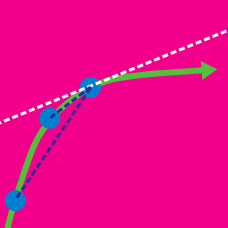Calculus

# Local Linear Approximation

If $f(1)=4$ and $f'(1)=6,$ which of the following is the best local linear approximation for $f(1.01)?$

Estimate the value of $\sqrt{0.979}$ using local linear approximation for the function $f(x)=\sqrt{1+x}.$

Use differentiation to estimate the value of $\sqrt{16.5}$.

Estimate the value of $\displaystyle \frac{6}{(1-x)^2}$ at $x=0.02$ using local linear approximation.

What is the local linear approximation of the function $f(x)=14x^3-5x$ near $x = 1?$

×